# Fluid Flow and Heat Transfer Analysis of Quadratic Free Convection in a Nanofluid Filled Porous Cavity

Fluid Flow and Heat Transfer Analysis of Quadratic Free Convection in a Nanofluid Filled Porous Cavity
Department of Basic and Applied Science, NIT Arunachal Pradesh, Yupia 791112, India

Corresponding Author Email:
vanavkumar.a@gmail.com
Page:
876-884
|
DOI:
https://doi.org/10.18280/ijht.390322
26 November 2020
|
Accepted:
15 February 2021
|
Published:
30 June 2021
| Citation

OPEN ACCESS

Abstract:

The involvement of non-linear convection effects in a natural convective fluid flow and heat transfer along with the effects of magnetic field in a porous cavity is studied numerically. Cu-water filled cavity of higher temperature at the left wall and lower temperature at the right wall. The governing equations are organized to achieve the required flow by using two-dimensional equations of energy, continuity and momentum. Vorticity-stream function based dimensionless equations are solved using the finite difference techniques. The results are discussed for various dimensionless parameters such as the Darcy number, non-linear convection parameter, Hartmann number, Rayleigh number and solid volume fraction of the nanoparticles. An augment in streamline velocity and convection heat transfer are observed by increasing the Rayleigh number, non-linear convection parameter and Darcy number. The non-linear convection parameter has a lesser effect on the lower Rayleigh numbers. Diminished streamline intensity and reduction in convection heat transfer are noted for an increase in the strength of the applied magnetic field irrespective of the non-linear convection parameter.

Keywords:

non-linear convection, porous, nanofluid, cavity, magnetic, heatlines

1. Introduction

Knowledge of understanding the natural convective fluid flow and heat transfer in a porous media is essential due to a bunch of applications in the area of crystal growth, underground energy storage, contaminant transportation, phase change energy storage devices, fuel cells, cooling of electronic modules and others. The modeling of heat and fluid flow occurs in a porous media and their necessities can be reviewed in the lectures [1-4]. Recently, a study on quadratic natural convective effect in a lid-driven cavity of the heated bottom wall is found in the literature .

Ghasemi et al.  discussed the effect of Hartmann number (magnetic field effect) over the flow occurs due to the natural convection in an Alumina-water nanofluid filled rectangular cavity. It is noted that the convection flow patterns are affected due to the effect of an applied magnetic field. Basak and Chamkha  analyzed a heatline flow that occurs by natural convection in various nanofluids filled square cavity with various types of thermal wall boundaries. The study explains a heat transfer of nanofluids, such as conduction or convection dominated heat transfer by using the heatlines or heatfunctions. Mahmoudi et al. [8, 9] discussed the Al2O3-water nanofluid flow and heat transfer in a square cavity due to natural convection subjected to a magnetic field. By quantification of applied magnetic field strength, it is observed that the suppression in flow intensity and reduction of convection heat transfer. Kobra et al.  and Rashad et al.  investigated the heat transfer of Cu-water nanofluid within a cavity addressed with the magnetic field effects. An investigation highlights that the convection heat transfer is reduced by increasing the Hartmann number between 0 to 50. Hartmann's number above 50 delivers a lesser affection against the convection heat transfer process.  Bondareva et al.  studied the heatline flow that occurs in an open-end cavity from the solid wall. The study identifies the reduction in the fluid flow and heat transfer due to fluid dynamic viscosity with the increase in the solid volume fraction of the nanoparticles. Rostami et al.  reviewed the control parameters available in the natural convective flow in a cavity of rectangular and non-rectangular shapes. Regardless of the fluid, the flow intensities and heat transfer are increased by increasing the Rayleigh number. The magnetic field, on the other hand, is involved in damping the effect of the flow velocity and therefore the magnetic field is cited as the flow controller. In addition to the above, the nanoparticles are involved in improving the heat transfer due to the increased thermal conductivity of the fluid.

Grosan et al.  investigated the inclination of the magnetic field on MHD free convection and internal heat generation effects in a rectangular porous cavity. Ahmed et al.  focused on modeling and study of natural convection in a right angle triangular shaped porous cavity. Fluid motion and temperature distributions are reduced due to an increase in the viscosity of the fluid by quantifying the solid volume fraction of the Cu nanoparticles. Ma et al.  considered a study on natural convection in a porous square cavity of three-sided cooled walls with varying cooling lengths in the vertical walls and heated at the bottom wall of the cavity. It is noted that an increase in the combinations of Rayleigh number and Darcy number increases the heat transfer by the convection. Nguyen et al.  analyzed the Cu-water nanofluid heat transfer by natural convection in a square porous cavity of a heated left wall. The flow due to convection is improved for higher Darcy number and stable or limitations in heat transfer are noticed for increasing the solid volume fraction of the nanoparticles. Balla et al.  discussed the behavior of MHD natural convective flow of various nanofluids in an inclined porous cavity. The study considers Al2O3, Cu, SiO2, TiO2 nanoparticles dispersed in water. Out of the above considered nanoparticles, Cu-water nanofluid model provides a higher heat transfer rate and TiO2-water nanofluid provides a lower heat transfer.  Mansour et al.  investigated the locations and size of the source and sink pairs in a porous enclosure filled with hybrid nanofluid. Investigation reveals that the Cu nanoparticles have a higher rate of heat transfer among other nanoparticles. Nguyen et al.  analyzed the flow of Cu-water nanofluid on a natural convective porous square cavity with a wavy interface. Sheikholeslami  investigated the MHD free convective effect in a porous, CuO-water nanofluid filled cavity. An increase in the Nusselt number is observed for the increase in Rayleigh number and solid volume fraction. And also, a decrease in the Nusselt number is noted for an increase in the Hartmann number. Al-Farhany and Abdulkadhim  studied the convective heat transfer from the conjugate wall of partially heated boundary to fluids in a porous cavity. Heat transfer from the conjugated wall is increased by increasing the Rayleigh number and the heat source length. Alhumoud  investigated natural convective heat transfer and fluid flow behavior due to the thermal boundaries present at the solid surfaces on either side of the porous cavity. An increase in convection heat transfer is noticed for increasing the Darcy number, thermal conductivity ratio of the wall and the Rayleigh number. Geridonmez and Oztop  applied a magnetic field in a porous cavity partially and studied its flow behavior. By applying the magnetic field partially, the heat transfer and fluid flow can be controlled as required. Belhadj et al.  studied the convection in a porous cavity using Darcy-Brinkman-Forcheimer formulae. It is noted that, enhancement of convective heat transfer is applicable for the increase in Darcy number and Rayleigh number, respectively. Bouafia et al.  investigated natural convective flows due to the corrugated heated and cooled boundary walls in a nanofluid filled porous cavity. The investigation shows an improvement in the heat transfer rate and reduction in recirculation of Al2O3-water nanofluid by increasing the solid volume fraction of the nanoparticles. Anandalakshmi et al.  analyzed the heat flow from the rhombic-shaped porous cavity subjected to the various boundary conditions. The heatline analysis provides an advantage in the visualization of heat propagation trajectories from the heated wall to the cold wall. Jino and Vanav [28, 29] investigated the MHD natural convective flows on Cu-water nanofluid filled square cavity of various boundary conditions in a saturated porous medium using the Kozey-Carman equation model. Majdi et al.  demonstrated the flows of nanofluids in the corrugated cavity, divided into parts with and without the porous layer along with the solid cylinder in between. The average Nusselt number increases with the increase in the Darcy number, Rayleigh number, solid volume fraction of the nanoparticles.

In the studies mentioned above, convective fluid flow and the heat transfer occur inside a porous cavity in the presence of an applied magnetic field with incorporating the non-linear convection effects is not yet considered. The study focuses on the importance of quadratic free convection when accuracy in the flow field is considered. The non-linear term involved in this research has to be considered on the effective modeling of free convection flow. A small variation in the flow field can give a greater impact on the performance of various applicational areas. Also in the above studies, it is concluded that the heat transfer of conventional fluids is increased by adding the nanoparticles into it, particularly Cu-water nanofluid. Hence, this study illustrates the flow of Cu-water nanofluid and heat transfer by the action of non-linear convection parameter on MHD natural convective in a porous cavity by using streamlines, isotherms and the heatlines.

2. Geometry and Mathematical Model

A geometry of equal spaced rectangle of each side length H, in x and y direction, is considered as shown in Figure 1. A non-dimensional temperature of θ=1 is distributed along the left wall of the cavity and θ=0 is distributed along the right wall of the cavity. Adiabatic temperature distribution of ∂θ/∂Y=0 is applied on both the horizontal walls. Each wall is immovable with zero velocities. Cu-water nanofluid (Prandtl number chosen as Pr=6.2) is filled in the entire porous domain. The nanoparticles (Cu) of size ranges between 1-100 nm is dispersed in a conventional fluid (water) is considered .

## 1.png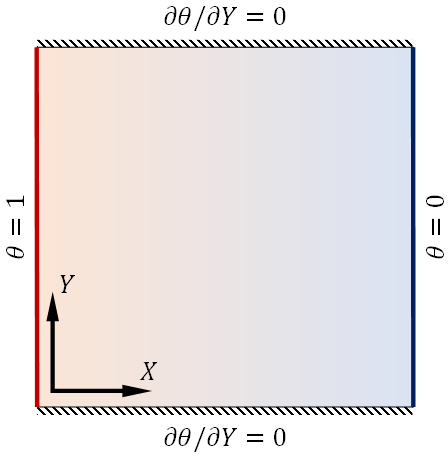Figure 1. Physical geometry of the problem

It is considered that the water and the nanoparticle (Cu) are in thermal equilibrium, the fluid is incompressible with laminar, dismission of radiation and viscous dissipation effects and applying the Boussinesq approximation. The density variation along with the temperature is denoted with the quadratic term . The temperature of the nanofluid flow and a porous bed is in thermal equilibrium and a nanofluid in the porous bed is designed using the Darcy–Brinkman model with neglecting the Forscheimer's inertia term in the momentum equation . Thus, the governing equations are generated to demonstrate the flow field of Cu-water nanofluid in a porous domain using above mentioned assumptions along with the influence of applied magnetic field on the horizontal direction of the cavity . The governing equations are written in terms of continuity, momentum and energy conservation equations on two-dimensional space.

$\frac{\partial u}{\partial x}+\frac{\partial v}{\partial y}=0$    (1)

$\rho_{n}\left[u \frac{\partial u}{\partial x}+v \frac{\partial u}{\partial y}\right]=\mu_{n}\left(\nabla^{2} \cdot \boldsymbol{u}\right)-S_{x}$    (2)

$\rho_{n}\left[u \frac{\partial v}{\partial x}+v \frac{\partial v}{\partial y}\right]=\mu_{n}\left(\nabla^{2} \cdot v\right)-S_{y}$    (3)

$\left(\rho c_{p}\right)_{n}\left[u \frac{\partial T}{\partial x}+v \frac{\partial T}{\partial y}\right]=k_{n}\left(\nabla^{2} \cdot \boldsymbol{T}\right)$    (4)

where, Sx=-∂p/∂x-u(μn/K) and $S_{y}=-\partial p / \partial y-v\left(\mu_{n} / K\right)-\sigma_{n} B^{2} v+(\rho \beta)_{n} g\left(T-T_{c}\right)+\left(\rho \beta^{*}\right)_{n} g\left(T-T_{c}\right)^{2}$ respectively. u and v are flow velocities along the x and y directions respectively. p is the pressure, μn is the nanofluids dynamic viscosity $\left(\mu_{n}=\mu_{f l} /(1-\phi)^{2.5}\right)$, ρn is the density of nanofluid $\left(\rho_{n}=(1-\phi) \rho_{f l}+\phi \rho_{p}\right)$, kn is the thermal conductivity of the nanofluid $\left(k_{n}=k_{f l}\left[\left[k_{p}+2 k_{f l}-2 \phi\left(k_{f l}-k_{p}\right)\right] /\left[k_{p}+2 k_{f l}-2 \phi\left(k_{f l}-k_{p}\right)\right]\right]\right)$, $\left(\rho c_{p}\right)_{n}$ is the heat capacitance of the nanofluid $\left(\left(\rho c_{p}\right)_{n}=(1-\phi)\left(\rho c_{p}\right)_{f l}+\phi\left(\rho c_{p}\right)_{p}\right)$, and (ρβ)n is the thermal expansion coefficient of the nanofluid $\left((\rho \beta)_{n}=(1-\phi)(\rho \beta)_{f l}+\phi(\rho \beta)_{p}\right)$ respectively  and their physical values are listed in Table 1.

Table 1. Physical properties

 Water Cu particle $\rho\left(\mathrm{kg} / \mathrm{m}^{3}\right)$ 997.1 8933 μ (Pa s) $8.9 \times 10^{-4}$ - $c_{p}(J / k g K)$ 4179 385 k (W/m K) 0.613 401 $\beta\left(K^{-1}\right)$ $2.1 \times 10^{-4}$ $16.7 \times 10^{-4}$

Using non-dimensional variables such as $X=x / H, Y=y / H, U=u H / \alpha_{f l}, V=v H / \alpha_{f l}, P=p H^{2} / \rho_{n} \alpha_{f l}^{2}$ and $\theta=\left(T-T_{C}\right) /\left(T_{h}-T_{c}\right)$ respectively. The dimensional governing Eqns. (1-4) are converted as dimensionless one as,

$\frac{\partial U}{\partial X}+\frac{\partial V}{\partial Y}=0$    (5)

$U \frac{\partial U}{\partial X}+V \frac{\partial U}{\partial Y}=\frac{\mu_{n}}{\rho_{n} \alpha_{f l}}\left(\frac{\partial^{2} U}{\partial X^{2}}+\frac{\partial^{2} U}{\partial Y^{2}}-\frac{U}{D a}\right)-\frac{\partial P}{\partial X}$    (6)

$U \frac{\partial V}{\partial X}+V \frac{\partial V}{\partial Y}=\frac{\mu_{n}}{\rho_{n} \alpha_{f l}}\left(\frac{\partial^{2} V}{\partial X^{2}}+\frac{\partial^{2} V}{\partial Y^{2}}-\frac{V}{D a}\right)-\frac{\partial P}{\partial Y}$

$\quad+\frac{(\rho \beta)_{n}}{\rho_{n} \beta_{f l}} R a \operatorname{Pr}\left(\theta+\gamma \theta^{2}\right)-H a^{2} \operatorname{Pr}(V)$    (7)

$U \frac{\partial \theta}{\partial X}+V \frac{\partial \theta}{\partial Y}=\frac{\alpha_{n}}{\alpha_{f l}}\left(\frac{\partial^{2} \theta}{\partial X^{2}}+\frac{\partial^{2} \theta}{\partial Y^{2}}\right)$    (8)

where,

Rayleigh number, $R a=\left(\left(T-T_{c}\right) \beta_{f l} g H^{3}\right) /\left(v_{f l} \alpha_{f l}\right)$

Darcy number, $D a=K / H^{2}$

Prandtl number, $P r=v_{f l} / \alpha_{f l}$

Non-linear convection parameter, $\gamma=\left(T_{h}-T_{c}\right) \beta^{*} / \beta_{f l}$

Hartmann number, $H a=\sqrt{\sigma_{n} / \rho_{n} v_{n}}(B H)$

Thermal diffusivity, $\alpha_{n}=k_{n} /\left(\rho c_{p}\right)_{n}$    (9)

The non-dimensional Eqns. (5-8) supported by no-slip boundary conditions (U=V=0) at all the boundaries and thermal boundaries of θ=1 at the left, θ=0 at the right and ∂θ/θY=0 at bottom and top of cavities with the maximum length of Xmax=Ymax=1 respectively.

Fluid flow, heat flow and heat transfer rate by a hot thermal boundary at the left wall of the cavity can be visualized by,

Streamlines, $\nabla^{2} \psi=\frac{\partial U}{\partial Y}-\frac{\partial V}{\partial X}$

Heatlines, $\nabla^{2} \Pi=\frac{\partial(U \theta)}{\partial Y}-\frac{\partial(V \theta)}{\partial X}$

Local Nusselt number, $N u_{l}=-\left(\frac{k_{n}}{k_{f l}}\right)\left(\frac{\partial \theta}{\partial X}\right)_{x=0}$

Average Nusselt number, $N u=\int N u_{l} d Y$    (10)

3. Numerical Solutions

Dimensionless governing Eqns. (5-8) can be modulated as vorticity, stream function based equations and which can be discretized and solved using finite difference techniques. The dependency of solutions on varying the number of grids for Da=10-2, Ra=105, ϕ=0.02, γ=0.5 and Ha=5 are illustrated in Figure 2. In the present study, a constant spacing grid of 122×122 computational domain is selected. A FORTRAN coding is designed to solve the governing equations and achieve the gallery of study visualization (10). Steady-state solutions are achieved at the required convergent criterion of running residuals (vorticity/temperature /velocities) below 10-5.

## 2.png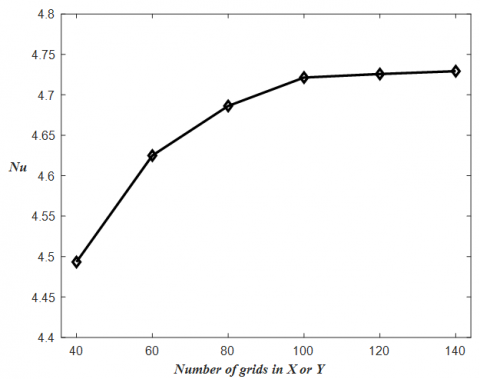Figure 2. Grid independence study

## 3.png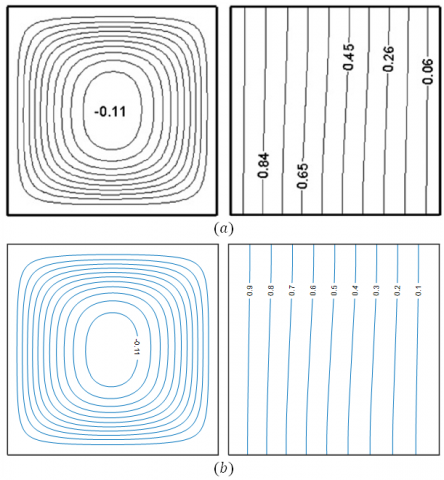Figure 3. Comparison of results by (a). Ghasemi et al.  and (b). Present work

Present work is initiated by validating the results obtained by designed code in FORTRAN and published work by Ghasemi et al. . The contours of streamlines and isotherms are good in comparison as shown in Figure 3.

4. Results and Discussion

Results and discussion involves the description of steady-state solutions using streamlines (ψ), isotherms (θ) and heatlines (Π) contours due to the combined quadratic natural convection and magnetic field in a porous cavity of square shaped fill up with the Cu-water nanofluid. The contours are specifically taken for the values of $\operatorname{Pr}(6.2)$, $R a\left(10^{3}, 10^{4}, 10^{5}, 10^{6}\right)$, $\gamma(0.0,0.5,1.0)$ $H a(0,5,25,50)$ and $D a\left(10^{-5}, 10^{-4}, 10^{-3}, 10^{-2}, 10^{-1}\right)$ respectively.

Figure 4 represents the contours of ψ, θ and Π in the absence of non-linear convection. The flow contours are given for the various values of Da at Ra=106, ϕ=0.02 and Ha=5. An increase in the streamlines flow circulation is found for changing the Da from 10-5 to 10-1. This is due to the effect of buoyancy force as a variation in permeability caused by changing the Da. The thermal boundary layer at higher Da causes the streamlines to more intense and covered with wider circulations. Inner core clockwise circulations of $\left|\psi_{\max }\right|=-0.65$ at lower Darcy number (Da=10-5) and $\left|\psi_{\max }\right|=-18$ at higher Darcy number (Da=10-1) are observed. Slight parallel temperature contour against vertical boundary gets inclined and later horizontal temperature lines are found in the isotherms contour for increasing the Da as 10-5, 10-4, 10-2 and 10-1. Heatlines circulations also get stronger for the increase in Da, which represents the convection dominated effects. At Da=10-5, the conduction is more dominated over the convection and for higher values of Darcy number (Da=10-1), convection is more dominated.

There is an increment in the flow intensities are observed by incorporating the non-linear convection effects. The contours for γ=0.5 is shown in Figure 5. ψmax values are increased for all the Da by varying from Da=10-5 to Da=10-1. At Da=10-1, there is an initiation of separation which is found in the circulation of the inner core. It is noted that an improvement in the temperature distribution and heatline circulation for all values of the Darcy number, which signifies an enhancement in the convection flow for γ=0.5 compared to the absence in the non-linear convection effects. Further increase of the non-linear convection parameter to γ=1.0, a further augment in the flow velocities and heatline circulations are noted in Figure 6. Flow initiated due to the proposed thermal boundary with heated left wall, causing circular/elliptical shaped clockwise rotation all over the cavity. The same nature of flow field is observed for various values of γ with improved heat transfer and circulation intensities. There are two separated circulations are observed in the center of the streamlines contour with ψmax=-22 for Da=10-1 and γ=1.0. Also, for larger value of the non-linear convection parameter at Da=10-5 the heatline circulations are improved.

## 4.png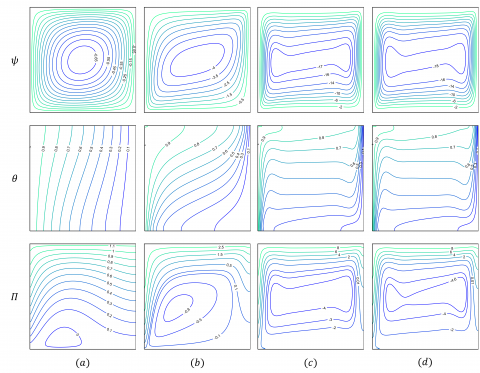Figure 4. Flow patterns for (a) Da=10-5, (b) Da=10-4, (c) Da=10-2, (d) Da=10-1 at Ra=106, ϕ=0.02, Ha=5 and γ=0.0

## 5.pngFigure 5. Flow patterns for (a) Da=10-5, (b) Da=10-4, (c) Da=10-2, (d) Da=10-1 at Ra=106, ϕ=0.02, Ha=5 and γ=0.5

## 6.png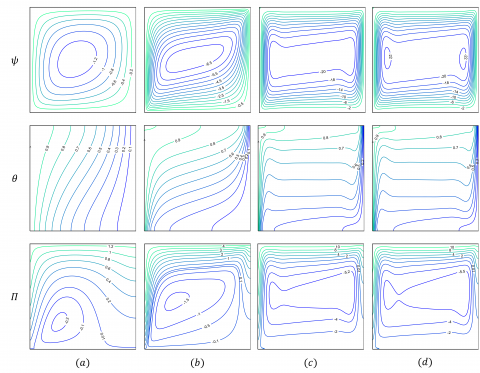Figure 6. Flow patterns for (a) Da=10-5, (b) Da=10-4, (c) Da=10-2, (d) Da=10-1 at Ra=106, ϕ=0.02, Ha=5 and γ=1.0

## 7.pngFigure 7. Flow patterns for (a) Da=103, (b) Da=104, (c) Da=105, (d) Ra=106 at Da=10-3, ϕ=0.02, Ha=5 and γ=0.5

Contours of ψ, θ, and Π for various values of Ra at Da=10-3, ϕ=0.02, γ=0.5 and Ha=5 are shown in Figure 7. The streamline with lesser circulation intensity, parallel nature of temperature distribution in isotherms and its orthogonally oriented heatlines of parallel to adiabatic walls indicates the clean conduction dominated heat transfer at Ra=103. Heat transfer from the left wall to the right wall is clearly demonstrated using the heatlines. An increased streamlines intensity and sharp bending of the temperature contour here indicates the buoyancy-driven effects, which is improved by increasing the Ra. The development of the thermal boundary layer and the higher heatlines circulation shows the convection heat transfer along the cavity. Also in the heatlines contour, denser lines near the higher temperature wall indicate convection dominated heat flow regime.

The effect of an applied magnetic field in a porous cavity in the presence of non-linear convection is illustrated in Figure 8. The figure shows the contours of streamlines, isotherms and heatlines for the values of Ra=106, Da=10-3, ϕ=0.02 and γ=0.5 Reduction in streamline circulations as ψmax=-14, -12 and -10 is observed in the case of increasing the Ha to 0,25, and 50. There is a similar kind of profiles are observed in the temperature contours for the above values of Ha. It is observed that the decrease in the intensity of the heatlines circulation and reduction in denser lines near the left wall of the heatlines contour by quantifying the Hartmann number, indicates the reduction of convection heat transfer effects.

Local Nusselt number obtained from the left wall of the cavity is shown in Figure 9. Nul is shown for different values of a non-linear parameter on Ra=103 and Ra=106 at Ra=10-3, Ha=5 and ϕ=0.02. Almost similar Nul is observed throughout the length of the cavity for lower Ra and reduction of Nul along the positive direction of the left wall is found in the cavity for higher Ra. Also, it is observed that, higher heat transfer at the bottom portion of the left wall. This is also denoted by the denser lines adjacent to the bottom-left of the isotherms contour (Figure 7). Reduction of local Nusselt number by increasing γ is found in the upper portion of the left wall and improvement of the local Nusselt number by increasing γ is found in the lower portion of the left wall at Ra=103. An enhancement of the Nul is observed by increasing the non-linear convection effect along the entire length of the left wall for higher Rayleigh number, Ra=106. This signifies the variation of the non-linear convection parameter has a minor effect on the lower Ra.

The average Nusselt number for various values of Da, γ, Ra for ϕ=0.02 and Ha=5 is shown in Figure 10. Nu increases for an increase in the Da and γ. Also, Nu increases for an increase in the Ra. It is noted that lesser differences in the Nusselt number for various values of γ at lower Darcy number (Da=10-5) on Ra=106 and at lower Rayleigh number (Ra=103) on Da=10-3. The variation of an average Nusselt number over various values of ϕ and Ha on considering the non-linear convection effects are illustrated in Figure 11. As before, Nu increases as the values of γ increases. A small rise in Nu is noted as the solid volume fraction of the nanoparticles increases, due to an improvement in the thermal conductivity of the nanofluid. The reduction of heat transfer is obtained with an increase in the values of Ha. The applied magnetic field acts in order to oppose the convective effect and causing the reduction in Nu.

## 8.png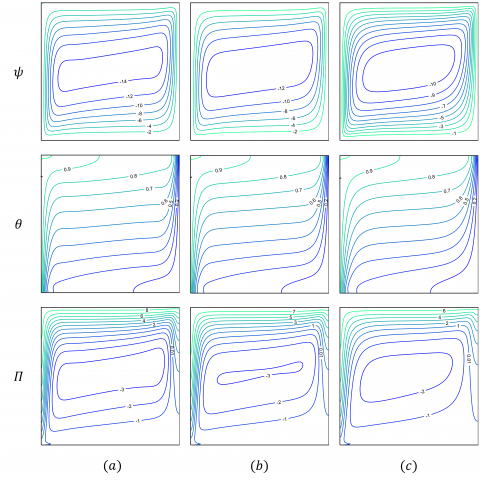Figure 8. Flow patterns for (a) Ha=0, (b) Ha=25, (c) Ha=50 at Ra=106, Da=10-3, ϕ=0.02 and γ=0.5

## 9.png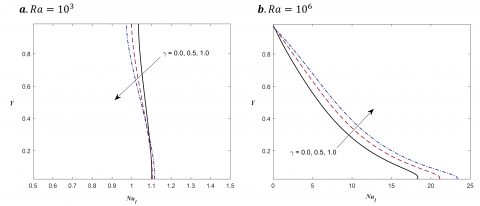Figure 9. Left wall distance vs local nusselt number for Da=10-3, Ha=5, ϕ=0.02

## 10.png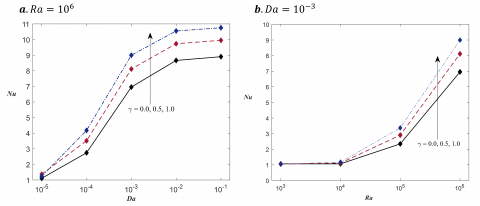Figure 10. Nu for ϕ=0.02 and Ha=5

## 11.png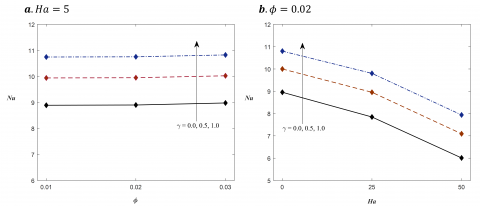Figure 11. Nu for Da=10-1 and Ra=106

5. Conclusions

The current work focuses on the incorporation of non-linearity effects on natural convection in a square-shaped porous cavity filled with the Cu-water nanofluid. Furthermore, the study includes the effects of the applied magnetic field on the horizontal direction in a porous cavity. The study is carried out for the various values of dimensionless parameters such as Da, Ha, γ, ϕ and Ra. The study includes the discussion of results using the heatlines, isotherms and streamlines.

Some of the findings observed in this study are, streamlines velocity is increased as an increasing function of Ra, Da, γ and a decreasing function of Ha. The heat transfer occurs from the left boundary to the right boundary in the cavity is clearly observed by using the heatlines. Additionally, the heatlines illustrate the conduction/convection heat transfer characteristics in the cavity. It is observed that the domination of convective heat transfer is significant by increasing Ra, Da and γ.

The introduction of non-linear convection behavior by increasing the value of γ leads to an increase in the convection heat transfer rate. The non-linear convection parameter has a lesser effect on the lower Rayleigh number and Darcy number. An improvement in the average Nusselt number is noticed for the higher values of Ra and Da. The effect of variation in heat transfer is less for increasing the amount of solid volume fraction of the nanoparticles. Finally, damping in the flow field and convection heat transfer is noticed with the augment in the applied magnetic field.

Acknowledgment

This work is supported by partial financial support through TEQIP-III Project, NIT Arunachal Pradesh and support through DST project, Sanction number: SERB ECR/2017/001007.

References

 Ingham, D.B., Pop, I. (1998). Transport Phenomena in Porous Media. Elsevier. https://doi.org/10.1016/B978-0-08-042843-7.X5000-4

 Bejan, A. (2006). Convection in Porous Media. In Convection in Porous Media. Springer New York. https://doi.org/10.1007/0-387-33431-9

 Vafai, K. (2015). Handbook of Porous Media. In K. Vafai (Ed.), Handbook of Porous Media. CRC Press. https://doi.org/10.1201/b18614

 Mansour, M.A., Rashad, A.M., Mallikarjuna, B., Hussein, A.K., Aichouni, M., Kolsi, L. (2019). MHD mixed bioconvection in a square porous cavity filled by gyrotactic microorganisms. International Journal of Heat and Technology, 37(2): 433-445. https://doi.org/10.18280/ijht.370209

 Venkatadri, K., Gouse Mohiddin, S., Suryanarayana Reddy, M. (2017). Hydromagneto quadratic natural convection on a lid driven square cavity with isothermal and non-isothermal bottom wall. Engineering Computations, 34(8): 2463-2478. https://doi.org/10.1108/EC-06-2017-0204

 Ghasemi, B., Aminossadati, S.M., Raisi, A. (2011). Magnetic field effect on natural convection in a nanofluid-filled square enclosure. International Journal of Thermal Sciences, 50(9): 1748-1756. https://doi.org/10.1016/j.ijthermalsci.2011.04.010

 Basak, T., Chamkha, A.J. (2012). Heatline analysis on natural convection for nanofluids confined within square cavities with various thermal boundary conditions. International Journal of Heat and Mass Transfer, 55(21-22): 5526-5543. https://doi.org/10.1016/j.ijheatmasstransfer.2012.05.025

 Mahmoudi, A., Mejri, I., Abbassi, M.A., Omri, A. (2014). Lattice boltzmann simulation of magnetic field direction effect on natural convection of nanofluid-filled cavity. International Journal of Heat and Technology, 32(1-2): 9-14. https://doi.org/10.18280/ijht.320102

 Mahmoudi, A., Mejri, I., Omri, A. (2016). Study of natural convection in a square cavity filled with nanofluid and subjected to a magnetic field. International Journal of Heat and Technology, 34(1): 73-79. https://doi.org/10.18280/ijht.340111

 Kobra, F., Quddus, N., Alim, M.A. (2014). Heat transfer enhancement of Cu-water nanofluid filled in a square cavity with a circular disk under a magnetic field. Procedia Engineering, Elsevier, 90: 582-587. https://dx.doi.org/10.1016/j.proeng.2014.11.776

 Rashad, A.M., Rashidi, M.M., Lorenzini, G., Ahmed, S.E., Aly, A.M. (2017). Magnetic field and internal heat generation effects on the free convection in a rectangular cavity filled with a porous medium saturated with Cu–water nanofluid. International Journal of Heat and Mass Transfer, 104: 878-889. http://dx.doi.org/10.1016/j.ijheatmasstransfer.2016.08.025

 Bondareva, N.S., Sheremet, M.A., Oztop, H.F., Abu-Hamdeh, N. (2017). Heatline visualization of natural convection in a thick walled open cavity filled with a nanofluid. International Journal of Heat and Mass Transfer, 109: 175-186. https://doi.org/10.1016/j.ijheatmasstransfer.2017.01.124

 Rostami, S., Aghakhani, S., Pordanjani, A.H., Afrand, M., Cheraghian, G., Oztop, H.F., Shadloo, M.S. (2020). A review on the control parameters of natural convection in different shaped cavities with and without nanofluid. Processes, 8(9): 1011. https://doi.org/10.3390/pr8091011

 Grosan, T., Revnic, C., Pop, I., Ingham, D.B. (2009). Magnetic field and internal heat generation effects on the free convection in a rectangular cavity filled with a porous medium. International Journal of Heat and Mass Transfer, 52(5-6): 1525-1533. https://doi.org/10.1016/j.ijheatmasstransfer.2008.08.011

 Ahmed, S.E., Rashad, A.M., Gorla, R.S.R. (2013). Natural convection in triangular enclosures filled with nanofluid saturated porous media. Journal of Thermophysics and Heat Transfer, 27(4): 700-706. https://doi.org/10.2514/1.T4029

 Belhadj, M., Atia, A., Benchatti, A. (2020). Analysis of natural convection in porous media for thermal storage using Darcy-Brinkman-Forcheimer formulation. Mathematical Modelling of Engineering Problems, 7(1): 73-78. https://doi.org/10.18280/mmep.070109

 Nguyen, M.T., Aly, A.M., Lee, S.W. (2015). Natural convection in a non-darcy porous cavity filled with Cu–water nanofluid using the characteristic-based split procedure in finite-element method. Numerical Heat Transfer; Part A: Applications, 67(2): 224-247. https://doi.org/10.1080/10407782.2014.923225

 Balla, C.S., Kishan, N., Gorla, R.S.R., Gireesha, B.J. (2017). MHD boundary layer flow and heat transfer in an inclined porous square cavity filled with nanofluids. Ain Shams Engineering Journal, 8(2): 237-254. https://doi.org/10.1016/j.asej.2016.02.010

 Mansour, M.A., Siddiqa, S., Gorla, R.S.R., Rashad, A.M. (2018). Effects of heat source and sink on entropy generation and MHD natural convection of Al2O3-Cu/water hybrid nanofluid filled with square porous cavity. Thermal Science and Engineering Progress, 6: 57-71. https://doi.org/10.1016/j.tsep.2017.10.014

 Nguyen, M.T., Aly, A.M., Lee, S.W. (2017). Effect of a wavy interface on the natural convection of a nanofluid in a cavity with a partially layered porous medium using the ISPH method. Numerical Heat Transfer, Part A: Applications, 72(1): 68-88. https://doi.org/10.1080/10407782.2017.1353385

 Sheikholeslami, M. (2017). CuO-water nanofluid free convection in a porous cavity considering Darcy law. European Physical Journal Plus, 132(1). https://doi.org/10.1140/epjp/i2017-11330-3

 Al-Farhany, K., Abdulkadhim, A. (2018). Numerical investigation of conjugate natural convection heat transfer in a square porous cavity heated partially from left sidewall. International Journal of Heat and Technology, 36(1): 237-244. https://doi.org/10.18280/ijht.360132

 Alhumoud, J.M. (2019). Non-equilibrium natural convection flow through a porous medium. Mathematical Modelling of Engineering Problems, 6(2): 163-169. https://doi.org/10.18280/mmep.060202

 Pekmen Geridonmez, B., Oztop, H.F. (2019). Natural convection in a cavity filled with porous medium under the effect of a partial magnetic field. International Journal of Mechanical Sciences, 161-162: 105077. https://doi.org/10.1016/j.ijmecsci.2019.105077

 Belhadj, M., Atia, A., Benchatti, A. (2020). Analysis of natural convection in porous media for thermal storage using Darcy-Brinkman-Forcheimer formulation. Mathematical Modelling of Engineering Problems, 7(1): 73–78. https://doi.org/10.18280/mmep.070109

 Bouafia, I., Mehdaoui, R., Kadri, S., Elmir, M. (2020). Natural convection in a porous cavity filled with nanofluid in the presence of isothermal corrugated source. International Journal of Heat and Technology, 38(2): 334-342. https://doi.org/10.18280/ijht.380208

 Anandalakshmi, R., Basak, T., Lukose, L. (2019). Numerical visualization via heatlines for natural convection in porous bodies of rhombic shapes subjected to thermal aspect ratio-based heating of walls. Numerical Heat Transfer, Part A: Applications, 76: 687-711. https://doi.org/10.1080/10407782.2019.1647734

 Jino, L., Vanav Kumar, A. (2020). Natural convection of water-cu nanofluid in a porous cavity with two pairs of heat source-sink and magnetic effect. International Journal of Mechanical and Production Engineering Research and Development, 10(3): 14481-14492. https://doi.org/10.24247/ijmperdjun20201378

 Jino, L., Vanav Kumar, A. (2020). Cu-water nanofluid natural convective heat and fluid flow in a porous cavity. International Journal of Mechanical and Production Engineering Research and Development, 10(3): 13695-13706. https://doi.org/10.24247/ijmperdjun20201305

 Majdi, H.S., Abdulkadhim, A., Abed, A.M. (2020). Computational fluid dynamics investigation of buoyancy driven flow between circular body and wavy enclosure filled with nanofluid/porous medium. International Journal of Heat and Technology, 38(2): 403-417. https://doi.org/10.18280/ijht.380216